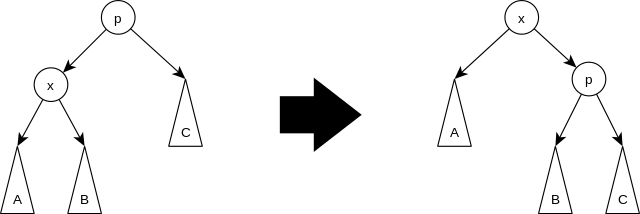# #456. 【UER #8】许愿树和圣诞树1. 点 $x$ 连续不断单旋向上，直至它成为点 $y$ 的儿子，保证 $y$ 是 $x$ 的祖先
2. 询问点 $x$ 的第 $k$ 级祖先，保证此时点 $x$ 的第 $k$ 级祖先存在
3. 询问整棵树先序遍历中的第 $k$ 个点，保证此时$1\le k \le n$。

### 样例一

#### input

10
2 3 5 3 9 8 6 5 0 9
10
3 9
1 1 2
2 1 4
1 4 5
1 4 5
2 1 3
1 2 4
2 8 1
2 10 1
2 8 2



#### output

7
9
4
5
9
9



### 限制与约定

$1$$1000$$0$$7 2$$100000$$0$$9$
$3$$100000$$1,2,3,4$$27 4$$100000$$2,3,4$$16$
$5$$100000$$3$$23 6$$100000$$5$$10$
$7$$100000$$8$